How to Perform Analyses of Variance in Excel - dummies

Someday, Excel’s Analysis ToolPak might have a choice labeled ANOVA: Mixed Design. That day, unfortunately, is not today. To run two ANOVAs on the same data and combine the ANOVA tables, follow these steps: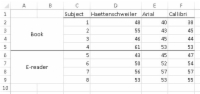## 1With the data entered into a worksheet, select DATA | Data Analysis.

The levels of the Between Group variable, Media (the A variable), are in the left column. The levels of the Within Group variable, Font (the B variable), are in the top row. Each cell entry is a reading speed in words per minute.

The Data Analysis dialog box opens.

## 2From the Data Analysis dialog box, select Anova: Two-Factor Without Replication.

This opens the Anova: Two-Factor Without Replication dialog box.

## 3In the Input Range box, enter the cell range that holds the data.

For this example, that’s C1:E9. This range includes the column headers, so select the Labels check box.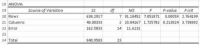## 4With the New Worksheet Ply radio button selected, click OK.

The result is the ANOVA table.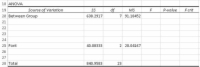## 5Modify the ANOVA table: Insert rows for terms from the second ANOVA, change names of the Sources of Variance, and delete unnecessary values.

First, insert four rows between Rows and Columns (between Row 20 and the original Row 21).

Next, change “Rows” to “Between Group” and “Columns” to “Font” (the name of the B variable).

Then, delete all the information from the row that has “Error” in the Source column.

Finally, delete the F-ratios, P-values and F-crits.

## 6Once again, select DATA | Data Analysis. This time, from the Data Analysis dialog box choose ANOVA: Two-Factor With Replication.

In the Input Range box, enter the cell array that holds the data, including the column headers. To do this, select C1:F9 in the worksheet.

## 7In the Rows Per Sample box, enter the number of subjects within each level of the Between Groups variable.

For this example, that’s 4. With the New Worksheet Ply radio button selected, click OK.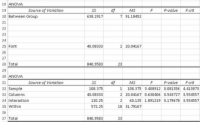## 8Copy the resulting ANOVA table and paste it into the worksheet with the first ANOVA, just below the first ANOVA table.

The worksheet should look like the one shown.

## 9Add Within Group to the first ANOVA table, four rows under Between Group, and calculate values for SS and df.

Type Within Group into row 24. The SS for Within Group is the SS Total – SS Between Group (B28-B20). The df for Within Group is the df Total – df Between Group (C28-C20).

## 10Copy the Sample row of data from the second ANOVA table and paste it into the first ANOVA table just below Between Group.

Copy and paste just the Source name (Sample), its SS and its df.

## 11Change “Sample” to the name of the Between Group variable (the A variable).

Change Sample to Media.

## 12In the next row, enter the name of the source for S/A, and calculate its SS, df, and MS.

Enter Subject/Media. The SS is the SS Between Group – SS Media (B20:B21). The df is the df Between Group – df Media (C20:C21). The MS is the SS divided by the df (B22/C22).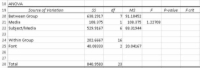## 13In the appropriate cell, calculate the F ratio for the A variable.

That’s MS Media divided by MS Subject/Media (D21/D22) calculated in E21.

## 14From the second ANOVA table, copy the Interaction, its SS, its df, and its MS, and paste into the first ANOVA table in the row just below the name of the B variable.

Change Interaction to the name of the interaction between the A variable and the B variable.

Copy the information from Row 34 into Row 26, just below Font. Change Interaction to Media X Font.

## 15In the next row, type the name of the source for B X S/A and calculate its SS, df, and MS.

Type Font X Subject/Media into A27. The SS is the SS Within Group – SS Font – SS Media X Font (B24 – B25 – B26). The df is the df Within Group – df Font – df Media X Font (C24 – C25 – C26). The MS is the SS divided by the df (B27/C27).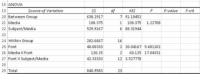## 16In the appropriate cells, calculate the remaining F-ratios.

In E25, divide D25 by D27. In E26, divide D26 by D27. For clarity, insert a row just above Total.

Use F.DIST.RT to find the P-values, and F.INV.RT to find the Fcrits. You can also delete the value for MSBetween Group, because it serves no purpose.